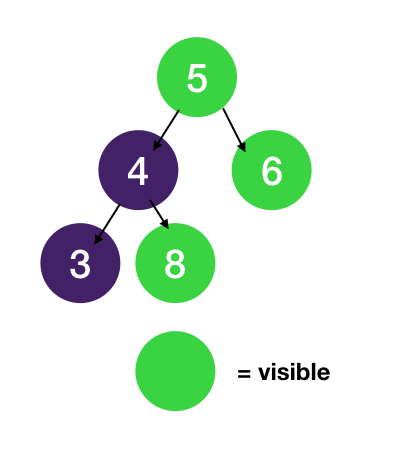Visible Tree Node | Number of Visible Nodes

Prereq: DFS on Tree

In a binary tree, we define a node "visible" when no node on the root-to-itself path (inclusive) has a greater value. The root is always "visible" since there are no other nodes between the root and itself. Given a binary tree, count the number of "visible" nodes.

Input:Output: 3

For example: Node 4 is not visible since 5>4, similarly Node 3 is not visible since both 5>3 and 4>3. Node 8 is visible since all 5<=8, 4<=8, and 8<=8.

Explanation

We can DFS on the tree and keep track of the max value we have seen as we go.

1. Decide on the return value

The problem asks for the total number of visible nodes, so we return the total number of visible nodes for the current subtree after we visit a node.

2. Identify states

The definition for a "visible" node is its value is greater than any other node's value on the root-to-itself path. To determine whether the current node is visible or not, we need to know the max value from the root to it. We can carry this as a state as we traverse down the tree.

Having decided on the state and return value we can now write the DFS.

Time Complexity: O(n)

There are n nodes and n - 1 edges in a tree so if we traverse each once then the total traversal is O(2n - 1) which is O(n).

1class Node:
2    def __init__(self, val, left=None, right=None):
3        self.val = val
4        self.left = left
5        self.right = right
6
7def visible_tree_node(root: Node) -> int:
8    def dfs(root, max_sofar):
9        if not root:
10            return 0
11
12        total = 0
13        if root.val >= max_sofar:
14            total += 1
15
16        total += dfs(root.left, max(max_sofar, root.val)) # max_sofar for child node is the larger of previous max and current node val
17        total += dfs(root.right, max(max_sofar, root.val))
18
20
21    # start max_sofar with smallest number possible so any value root has is smaller than it
22    return dfs(root, -float('inf'))
23
24def build_tree(nodes, f):
25    val = next(nodes)
26    if val == 'x': return None
27    left = build_tree(nodes, f)
28    right = build_tree(nodes, f)
29    return Node(f(val), left, right)
30
31if __name__ == '__main__':
32    root = build_tree(iter(input().split()), int)
33    res = visible_tree_node(root)
34    print(res)
35